Do you like the article?
Share it with others -
Use new possibilities of MetaTrader 5

# Processing optimization results using the graphical interface

12 July 2018, 12:03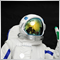0
12 695

### Introduction

This is a continuation of the idea of processing and analysis of optimization results. The previous article contained the description of the way to visualize optimization results using the MQL5 application graphical interface. This time, the task is more complicated: we will choose 100 best optimization results and display them in the graphical interface table.

In addition, we continue to develop the idea of multi-symbol balance graphs, which was also presented in a separate article. Let us combine the ideas of these two articles and enable the user to select a row in the optimization results table and receive a multi-symbol balance and drawdown graph on separate charts. After optimizing Expert Advisor parameters, the trader will be able to perform fast analysis of results and choose appropriate values to work with.

### Developing the graphical interface

The GUI of the test Expert Advisor will consist of the following elements.

• Form for controls
• Status bar for displaying additional summary information
• Tabs for arranging elements in groups:
• Frames
• Input field for managing the number of displayed balance results while re-scrolling results after optimization
• Delay in milliseconds while scrolling results
• Button to start re-scrolling of results
• Graph of the specified number of balance results
• Graph of all results
• Results
• Table of best results
• Balance
• Multi-symbol balance graph for the result selected in the table
• Drwadown graph for the result selected in the table
• Indication for the frame replaying process

The code of methods for creating elements listed above is available as a separate include file for use with the MQL program class:

```//+------------------------------------------------------------------+
//| Class for creating an application                                |
//+------------------------------------------------------------------+
class CProgram : public CWndEvents
{
private:
//--- Window
CWindow           m_window1;
//--- Status Bar
CStatusBar        m_status_bar;
//--- Tabs
CTabs             m_tabs1;
//--- Edits
CTextEdit         m_curves_total;
CTextEdit         m_sleep_ms;
//--- Buttons
//--- Charts
CGraph            m_graph1;
CGraph            m_graph2;
CGraph            m_graph3;
CGraph            m_graph4;
//--- Tables
CTable            m_table_param;
//--- Progress bar
CProgressBar      m_progress_bar;
//---
public:
//--- Create the graphical interface
bool              CreateGUI(void);
//---
private:
//--- Form
bool              CreateWindow(const string text);
//--- Status Bar
bool              CreateStatusBar(const int x_gap,const int y_gap);
//--- Tabs
bool              CreateTabs1(const int x_gap,const int y_gap);
//--- Edits
bool              CreateCurvesTotal(const int x_gap,const int y_gap,const string text);
bool              CreateSleep(const int x_gap,const int y_gap,const string text);
//--- Buttons
bool              CreateReplyFrames(const int x_gap,const int y_gap,const string text);
//--- Charts
bool              CreateGraph1(const int x_gap,const int y_gap);
bool              CreateGraph2(const int x_gap,const int y_gap);
bool              CreateGraph3(const int x_gap,const int y_gap);
bool              CreateGraph4(const int x_gap,const int y_gap);
//--- Buttons
bool              CreateUpdateGraph(const int x_gap,const int y_gap,const string text);
//--- Tables
bool              CreateMainTable(const int x_gap,const int y_gap);
//--- Progress bar
bool              CreateProgressBar(const int x_gap,const int y_gap,const string text);
};
//+------------------------------------------------------------------+
//| Methods for creating controls                                    |
//+------------------------------------------------------------------+
#include "CreateGUI.mqh"
//+------------------------------------------------------------------+
```

As mentioned above, the table will display 100 best optimization results (in terms of the largest final profit). Since the GUI is created before the optimization start, the table is initially empty. The number of columns and text for headers is determined in the optimization frame processing class.

Let us create a table with the following set of functions.

• Sorting option
• Selection of a row
• Fixing of a selected row (without the ability to deselect)
• Manual adjustment of column width
• Formatting in Zebra style

The code for creating the table is shown below. To have the table fixed in the second tab, the table object should be passed to the tabs object with the indication of the tab index. In this case, the table's main class is the 'Tabs' element. Thus, if the size of the tab area is changed, the table size will change relative to its main element, provided that this is specified in 'Table' element properties.

```//+------------------------------------------------------------------+
//| Create the main table                                            |
//+------------------------------------------------------------------+
bool CProgram::CreateMainTable(const int x_gap,const int y_gap)
{
//--- Store the pointer to the main control
m_table_param.MainPointer(m_tabs1);
//--- Attach to tab
//--- Properties
m_table_param.TableSize(1,1);
m_table_param.IsSortMode(true);
m_table_param.SelectableRow(true);
m_table_param.IsWithoutDeselect(true);
m_table_param.ColumnResizeMode(true);
m_table_param.IsZebraFormatRows(clrWhiteSmoke);
m_table_param.AutoXResizeMode(true);
m_table_param.AutoYResizeMode(true);
m_table_param.AutoXResizeRightOffset(2);
m_table_param.AutoYResizeBottomOffset(2);
//--- Create a control
if(!m_table_param.CreateTable(x_gap,y_gap))
return(false);
//--- Add the object to the common array of object groups
return(true);
}
```

### Saving optimization results

The CFrameGenerator class is implemented for working with optimization results. We will use a version from the article Visualizing trading strategy optimization in MetaTrader 5 and will add necessary methods to it. In addition to saving the total balance and final statistics in frames, we need to save balance and drawdown for each symbol separately. The separate array structure CSymbolBalance will be used for saving balances. The structure has a dual purpose. Data saved to its arrays will then be passed to a frame in a common array. After optimization, data will be extracted from the frame array and passed back to the arrays of this structure, to be displayed on multi-symbol balance graphs.

```//--- Array for all symbol balances
struct CSymbolBalance
{
double            m_data[];
};
//+------------------------------------------------------------------+
//| Class for working with optimization results                      |
//+------------------------------------------------------------------+
class CFrameGenerator
{
private:
//--- Structure of balances
CSymbolBalance    m_symbols_balance[];
};
```

The enumeration of symbols separated by ',' will be passed to the frame as a string parameter. Initially, data were supposed to be saved to a frame as a full report in a string array. But string arrays cannot be passed to a frame at the moment. An attempt to pass a string array to the FrameAdd() function will produce an error during compilation:

string arrays and structures containing objects are not allowed

Another option is to write the report to a file and pass this file to the frame. However, this option is not suitable: we would have to record the results to a hard disk too often.

Therefore, I decided to collect all the necessary data into one array and then extract data based on keys contained in the frame parameters. Statistical variables will be contained at the very beginning of the array. This will be followed by the total balance and separate balance values per symbols. Drawdown data for two axes separately will be located at the end.

The below scheme shows the order of data packing in the array. A variant with two symbols is shown to keep the scheme short enough.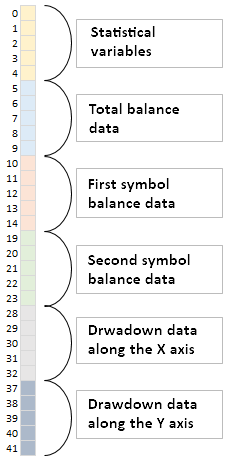Fig. 1. Sequence of data arrangement in the array.

So, we need keys for determining indices of each range in the array. The number of statistical variables is constant and determined in advance. We will display in the table five variables and a pass number to ensure that the data of this result can be accessed after optimization:

```//--- Number of statistical parameters
#define STAT_TOTAL 6
```

The amount of balance data will be the same for the total data and individual symbol data. This value will be sent to the FrameAdd() function as a double parameter. To determine symbols used in testing, we will define them during each pass, in the OnTester() function based on the history of trades. This information will be sent to the FrameAdd() function as a string parameter.

`::FrameAdd(m_report_symbols,1,data_count,stat_data);`

The sequence of symbols specified in the string parameter matches data sequence in the array. Thus, having all these parameters, we can properly extract data packed into the array.

The CFrameGenerator::GetHistorySymbols() method for determining symbols in the history of deals is presented in the code below:

```#include <Trade\DealInfo.mqh>
//+------------------------------------------------------------------+
//| Class for working with optimization results                      |
//+------------------------------------------------------------------+
class CFrameGenerator
{
private:
CDealInfo         m_deal_info;
//--- Symbols from the report
string            m_report_symbols;
//---
private:
//--- Get symbols from the account history and return their number
int               GetHistorySymbols(void);
};
//+------------------------------------------------------------------+
//| Get symbols from the account history and return their number     |
//+------------------------------------------------------------------+
int CFrameGenerator::GetHistorySymbols(void)
{
//--- Go through the loop for the first time and get traded symbols
int deals_total=::HistoryDealsTotal();
for(int i=0; i<deals_total; i++)
{
//--- Get the deal ticket
if(!m_deal_info.SelectByIndex(i))
continue;
//--- If there is a symbol name
if(m_deal_info.Symbol()=="")
continue;
//--- If there is no such a string, add it
if(::StringFind(m_report_symbols,m_deal_info.Symbol(),0)==-1)
}
//--- Get string elements by separator
ushort u_sep=::StringGetCharacter(",",0);
int symbols_total=::StringSplit(m_report_symbols,u_sep,m_symbols_name);
//--- Return the number of symbols
return(symbols_total);
}
```

If the history of deals contains more than one symbol, the array size is increased by one. The first element is reserved for the total balance.

```//--- Set the balance array size by the number of symbols + 1 for the total balance
::ArrayResize(m_symbols_balance,(m_symbols_total>1)? m_symbols_total+1 : 1);
```

Once all data from the history of deals are saved to separate arrays, they should be placed in one common array. The CFrameGenerator::CopyDataToMainArray() method is used for that purpose. Here, we sequentially increase the common array by the amount of added data, in a cycle. Then, during the last iteration, we copy the drawdown.

```class CFrameGenerator
{
private:
//--- Result balance
double            m_balances[];
//---
private:
//--- Copies balance data to the main array
void              CopyDataToMainArray(void);
};
//+------------------------------------------------------------------+
//| Copies balance data to the main array                            |
//+------------------------------------------------------------------+
void CFrameGenerator::CopyDataToMainArray(void)
{
//--- Number of balance curves
int balances_total=::ArraySize(m_symbols_balance);
//--- Balance array size
int data_total=::ArraySize(m_symbols_balance.m_data);
//--- Fill the common array with data
for(int i=0; i<=balances_total; i++)
{
//--- The current balance amount
int array_size=::ArraySize(m_balances);
//--- Copy balance values to the array
if(i<balances_total)
{
//--- Copy balance to the array
::ArrayResize(m_balances,array_size+data_total);
::ArrayCopy(m_balances,m_symbols_balance[i].m_data,array_size);
}
//--- Copy drawdown values to the array
else
{
data_total=::ArraySize(m_dd_x);
::ArrayResize(m_balances,array_size+(data_total*2));
::ArrayCopy(m_balances,m_dd_x,array_size);
::ArrayCopy(m_balances,m_dd_y,array_size+data_total);
}
}
}
```

Statistical variables are added at the beginning of the common array, in the CFrameGenerator::GetStatData() method. The array, which will eventually be saved in the frame, is passed to this method by reference. Its size is set as the balance data array size plus the number of statistical variables. Balance data are placed from the last index in the range of statistical variables.

```class CFrameGenerator
{
private:
//--- Get statistical data
void              GetStatData(double &dst_array[],double on_tester_value);
};
//+------------------------------------------------------------------+
//| Get statistical data                                             |
//+------------------------------------------------------------------+
void CFrameGenerator::GetStatData(double &dst_array[],double on_tester_value)
{
//--- Copy the array
::ArrayResize(dst_array,::ArraySize(m_balances)+STAT_TOTAL);
::ArrayCopy(dst_array,m_balances,STAT_TOTAL,0);
//--- Fill in the first values of the array (STAT_TOTAL) with the test results
dst_array =0;                                             // номер прохода
dst_array =on_tester_value;                               // value of the custom optimization criterion
dst_array =::TesterStatistics(STAT_PROFIT);               // net profit
dst_array =::TesterStatistics(STAT_EQUITY_DDREL_PERCENT); // maximum drawdown in %
dst_array =::TesterStatistics(STAT_RECOVERY_FACTOR);      // recovery factor
}
```

The above described actions are performed in the CFrameGenerator::OnTesterEvent() method, which is called in the main program file, in the OnTester() function.

```//+------------------------------------------------------------------+
//| Prepares an array of balance values and sends it in a frame      |
//| The function should be called in the EA in the OnTester() handler|
//+------------------------------------------------------------------+
void CFrameGenerator::OnTesterEvent(const double on_tester_value)
{
//--- Get balance data
int data_count=GetBalanceData();
//--- Array for sending data to a frame
double stat_data[];
GetStatData(stat_data,on_tester_value);
//--- Create a data frame and send it to the terminal
::Print(__FUNCTION__," > Frame add error: ",::GetLastError());
else
}
```

The table arrays will be filled at the end of optimization, in the FinalRecalculateFrames() method, which is called in the CFrameGenerator::OnTesterDeinitEvent() method. The following actions are performed here: the final recalculation of optimization results, determining of the number of optimized parameters, filling of the array of table headers, collection of data to to table arrays. After that data are sorted by the specified criteria.

Let's consider some auxiliary methods, which will be called in the final frame processing cycle. Let's start with CFrameGenerator::GetParametersTotal(), which determines the number of EA parameters used in the optimization.

FrameInputs() function is called for obtaining Expert Advisor parameters from the frame. By passing the pass number to this function, we can get an array of parameters and their number. Parameters used in optimization are listed first, then other parameters are indicated. Only optimization parameters will be shown in the table, therefore we need to determine the index of the first unoptimized parameter - this will help us remove the group, which should not be included in the table. We can specify the first unoptimized external EA parameter in advance, which the program will use. In this case, this is Symbols. Knowing the index, we can calculate the number of Expert Advisor optimization parameters.

```class CFrameGenerator
{
private:
//--- The first unoptimized parameter
string            m_first_not_opt_param;
//---
private:
//--- Get the number of optimization parameters
void              GetParametersTotal(void);
};
//+------------------------------------------------------------------+
//| Constructor                                                      |
//+------------------------------------------------------------------+
CFrameGenerator::CFrameGenerator(void) : m_first_not_opt_param("Symbols")
{
}
//+------------------------------------------------------------------+
//| Get the number of optimization parameters                        |
//+------------------------------------------------------------------+
void CFrameGenerator::GetParametersTotal(void)
{
//--- In the first frame, determine the number of optimization parameters
if(m_frames_counter<1)
{
//--- Get the input parameters of the Expert Advisor, for which the frame is formed
::FrameInputs(m_pass,m_param_data,m_par_count);
//--- Find the index of the first unoptimized parameter
int limit_index=0;
int params_total=::ArraySize(m_param_data);
for(int i=0; i<params_total; i++)
{
if(::StringFind(m_param_data[i],m_first_not_opt_param)>-1)
{
limit_index=i;
break;
}
}
//--- The number of optimization parameters
m_param_total=(m_par_count-(m_par_count-limit_index));
}
}
```

The table data will be stored in the CReportTable array structure. After we found the number of EA optimization parameters, we can determine and set the number of columns for the table. This is done in the CFrameGenerator::SetColumnsTotal() method. The number of rows is initially equal to zero

```//--- Table arrays
struct CReportTable
{
string            m_rows[];
};
//+------------------------------------------------------------------+
//| Class for working with optimization results                      |
//+------------------------------------------------------------------+
class CFrameGenerator
{
private:
//--- Report table
CReportTable      m_columns[];
//---
private:
//--- Set the number of table columns
void              SetColumnsTotal(void);
};
//+------------------------------------------------------------------+
//| Set the number of table columns                                  |
//+------------------------------------------------------------------+
void CFrameGenerator::SetColumnsTotal(void)
{
//--- Determine the number of columns for the results table
if(m_frames_counter<1)
{
int columns_total=int(STAT_TOTAL+m_param_total);
::ArrayResize(m_columns,columns_total);
for(int i=0; i<columns_total; i++)
::ArrayFree(m_columns[i].m_rows);
}
}
```

Rows are added in the CFrameGenerator::AddRow() method. In the process of working with frames, only results having trades will be added to the table. The first columns of the table will show the pass number, statistical variables and then Expert Advisor optimization parameters. When parameters are obtained from a frame, they are available in the format "parameterN=valueN" [parameter name][separator][parameter value]. We need only parameter values, which should be added to the table. Therefore, let's split the line according to the separator ‘=’, and save the value from the second element of the array.

```class CFrameGenerator
{
private:
};
//+------------------------------------------------------------------+
//| Add a data row                                                   |
//+------------------------------------------------------------------+
{
//--- Set the number of columns in the table
SetColumnsTotal();
//--- Exit if there are no trade
if(m_data<1)
return;
//--- Fill the table
int columns_total=::ArraySize(m_columns);
for(int i=0; i<columns_total; i++)
{
int prev_rows_total=::ArraySize(m_columns[i].m_rows);
::ArrayResize(m_columns[i].m_rows,prev_rows_total+1,RESERVE);
//--- Pass number
if(i==0)
{
m_columns[i].m_rows[prev_rows_total]=string(m_pass);
continue;
}
//--- Statistical parameters
if(i<STAT_TOTAL)
m_columns[i].m_rows[prev_rows_total]=string(m_data[i]);
//--- EA optimization parameters
else
{
string array[];
if(::StringSplit(m_param_data[i-STAT_TOTAL],'=',array)==2)
m_columns[i].m_rows[prev_rows_total]=array;
}
}
}
```

Table headers are taken using the special method CFrameGenerator::GetHeaders() - the first element of the array elements in the split line:

```class CFrameGenerator
{
private:
//--- Get headers for the table
};
//+------------------------------------------------------------------+
//| Get headers for the table                                        |
//+------------------------------------------------------------------+
{
int columns_total =::ArraySize(m_columns);
for(int c=STAT_TOTAL; c<columns_total; c++)
{
string array[];
if(::StringSplit(m_param_data[c-STAT_TOTAL],'=',array)==2)
}
}
```

Let's use the simple method CFrameGenerator::ColumnSortIndex() to inform the program what criterion to use in order to choose 100 optimization results for the table. The column index is passed to the method. After the end of optimization, the results table will be sorted by this index in descending order, and the top 100 results will be included in the table and displayed in the graphical interface. The third column (index 2) is set by default, i.e. the results will be sorted by the maximum profit.

```class CFrameGenerator
{
private:
//--- The index of the sorted table
uint              m_column_sort_index;
//---
public:
//--- Set the index of the column, by which the table will be sorted
void              ColumnSortIndex(const uint index) { m_column_sort_index=index; }
};
//+------------------------------------------------------------------+
//| Constructor                                                      |
//+------------------------------------------------------------------+
CFrameGenerator::CFrameGenerator(void) : m_column_sort_index(2)
{
}
```

If you need to pick up results based on another criterion, CFrameGenerator::ColumnSortIndex() should be called in the CProgram::OnTesterInitEvent() method at the very beginning of the optimization:

```//+------------------------------------------------------------------+
//| Optimization process start event                                 |
//+------------------------------------------------------------------+
void CProgram::OnTesterInitEvent(void)
{
...
m_frame_gen.ColumnSortIndex(3);
...
}
```

As a result, the CFrameGenerator::FinalRecalculateFrames() method for the final recalculation of frames now works according to the following algorithm.

• Move the frame pointer to the list beginning. Reset the counter of frames and the arrays.
• Iterate over all frames in a loop and:
• get the number of optimization parameters,
• distribute negative and positive results in arrays,
• add a data row to the table.
• After the frame iteration cycle, get the table headers.
• Then sort the table by the column specified in settings.
• The method is completed by the update of the optimization results graph.

Code of CFrameGenerator::FinalRecalculateFrames():

```class CFrameGenerator
{
private:
//--- Final recalculation of data from all frames after optimization
void              FinalRecalculateFrames(void);
};
//+------------------------------------------------------------------+
//| Final recalculation of data from all frames after optimization   |
//+------------------------------------------------------------------+
void CFrameGenerator::FinalRecalculateFrames(void)
{
//--- Move the frame pointer to the beginning
::FrameFirst();
//--- Reset the counter and the arrays
ArraysFree();
m_frames_counter=0;
//--- Start going through frames
while(::FrameNext(m_pass,m_name,m_id,m_value,m_data))
{
//--- Get the number of optimization parameters
GetParametersTotal();
//--- Negative result
if(m_data[m_profit_index]<0)
//--- Positive result
else
//--- Increase the counter of processed frames
m_frames_counter++;
}
//--- Get headers for the table
//--- The number of rows and columns
int rows_total =::ArraySize(m_columns.m_rows);
//--- Sort the table by the specified column
QuickSort(0,rows_total-1,m_column_sort_index);
//--- Update the series on the chart
CCurve *curve=m_graph_results.CurveGetByIndex(0);
curve.Name("P: "+(string)ProfitsTotal());
curve.Update(m_profit_x,m_profit_y);
//---
curve=m_graph_results.CurveGetByIndex(1);
curve.Name("L: "+(string)LossesTotal());
curve.Update(m_loss_x,m_loss_y);
//--- Horizontal axis properties
CAxis *x_axis=m_graph_results.XAxis();
x_axis.Min(0);
x_axis.Max(m_frames_counter);
x_axis.DefaultStep((int)(m_frames_counter/8.0));
//--- Refresh the graph
m_graph_results.CalculateMaxMinValues();
m_graph_results.CurvePlotAll();
m_graph_results.Update();
}
```

Next, let's consider the methods used to receive data from a frame upon a request from the user.

### Extracting data from a frame

We have considered the structure of a common array with the sequence of data of different categories. Now we need to understand how data are extracted from this array. Frames contain the size of balance arrows and the enumeration of symbols as keys. If the size of balance arrays were equal to the size of drawdown arrays, then we could be able to determine the indices of all the ranges of packed data by a single formula, in a cycle, as in the scheme below. But the sizes of the arrays are different. Therefore, during the last iteration in the cycle, we need to determine how many elements are left in the data range that relates to drawdowns, and divide it by two, since the sizes of the drawdown arrays are equal.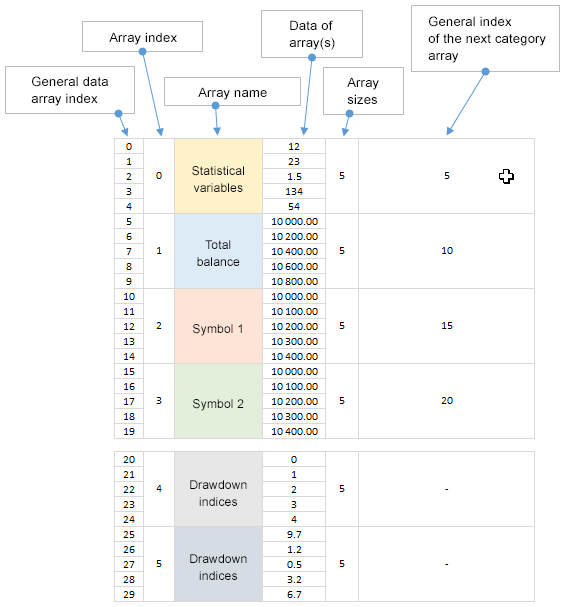Fig. 2. A scheme with parameters for calculating the index of the array from the next category.

The public method CFrameGenerator::GetFrameData() is implemented for obtaining data from a frame. Let's consider it in more detail.

At the beginning of the method, we need to move the frame pointer to the list beginning. After that the process of iteration of all frames with optimization results begins. We need to find the frame, the pass number of which was passed to the method as an argument. If it is found, the program continues to work according to the following algorithm.

• The size of the common array with the frame data is obtained.
• Elements of the string parameter row and the number of such elements are obtained. If there are more than one symbol, the number of balances in the array is increased by one. Thus, the first range is the total balance, other ranges apply to balances by symbols.
• Next, data need to be moved to arrays of balances. We run a cycle to extract data from the common array (the number of iterations is equal to the number of balances). To determine the first index to start copying data at, we make a shift by the number of statistical variables (STAT_TOTAL) and multiply the iteration index (i) by the size of the balance array (m_value). Thus, during each iteration we get data of all balances into separate arrays.
• During the last iteration, we get drawdown data into separate arrays. These are the last data in the array, so we only need to find out the remaining number of elements and divide it by 2. Next, in two consecutive steps we obtain drawdown data
• The last step is to refresh graphs by applying new data and stop the frame iteration cycle.
```class CFrameGenerator
{
public:
//--- Get data according to the specified frame number
void              GetFrameData(const ulong pass_number);
};
//+------------------------------------------------------------------+
//| Get data according to the specified frame number                 |
//+------------------------------------------------------------------+
void CFrameGenerator::GetFrameData(const ulong pass_number)
{
//--- Move the frame pointer to the beginning
::FrameFirst();
//--- Extract data
while(::FrameNext(m_pass,m_name,m_id,m_value,m_data))
{
//--- Pass numbers do not match, move to the next
if(m_pass!=pass_number)
continue;
//--- The size of the data array
int data_total=::ArraySize(m_data);
//--- Get string elements by separator
ushort u_sep          =::StringGetCharacter(",",0);
int    symbols_total  =::StringSplit(m_name,u_sep,m_symbols_name);
int    balances_total =(symbols_total>1)? symbols_total+1 : symbols_total;
//--- Set size for the array of the number of balances
::ArrayResize(m_symbols_balance,balances_total);
//--- Distribute data between arrays
for(int i=0; i<balances_total; i++)
{
//--- Free the data array
::ArrayFree(m_symbols_balance[i].m_data);
//--- Define the index, copying of source data is to start from
int src_index=STAT_TOTAL+int(i*m_value);
//--- Copy data to the array of balances structure
::ArrayCopy(m_symbols_balance[i].m_data,m_data,0,src_index,(int)m_value);
//--- If this is the last iteration, get the data of drawdowns
if(i+1==balances_total)
{
//--- Get the amount of remaining data and the size for arrays along two axes
double dd_total   =data_total-(src_index+(int)m_value);
double array_size =dd_total/2.0;
//--- Index to start copying from
src_index=int(data_total-dd_total);
//--- Set size for the array of drawdowns
::ArrayResize(m_dd_x,(int)array_size);
::ArrayResize(m_dd_y,(int)array_size);
//--- Sequentially copy data
::ArrayCopy(m_dd_x,m_data,0,src_index,(int)array_size);
::ArrayCopy(m_dd_y,m_data,0,src_index+(int)array_size,(int)array_size);
}
}
//--- Refresh graphs and stop the cycle
UpdateMSBalanceGraph();
UpdateDrawdownGraph();
break;
}
}
```

To obtain data from the cells of the table array, we call the CFrameGenerator::GetValue() public method, specifying the index of the table column and row in its arguments.

```class CFrameGenerator
{
public:
//--- Returns a value from the specified cell
string            GetValue(const uint column_index,const uint row_index);
};
//+------------------------------------------------------------------+
//| Returns a value from the specified cell                          |
//+------------------------------------------------------------------+
string CFrameGenerator::GetValue(const uint column_index,const uint row_index)
{
//--- Checking for exceeding the column range
uint csize=::ArraySize(m_columns);
if(csize<1 || column_index>=csize)
return("");
//--- Checking for exceeding the row range
uint rsize=::ArraySize(m_columns[column_index].m_rows);
if(rsize<1 || row_index>=rsize)
return("");
//---
return(m_columns[column_index].m_rows[row_index]);
}
```

### Data visualization and interaction with the graphical interface

Two more objects of the CGraphic type are declared in the CFrameGenerator class for refreshing charts by applying balance and drawdown data. Like with other objects of the same type in CFrameGenerator, we need to pass pointers to GUI elements in them, to the CFrameGenerator::OnTesterInitEvent() method at the very beginning of optimization.

```#include <Graphics\Graphic.mqh>
//+------------------------------------------------------------------+
//| Class for working with optimization results                      |
//+------------------------------------------------------------------+
class CFrameGenerator
{
private:
//--- Pointers to graphs for data visualization
CGraphic         *m_graph_ms_balance;
CGraphic         *m_graph_drawdown;
//---
public:
//--- Strategy tester events handlers
void              OnTesterInitEvent(CGraphic *graph_balance,CGraphic *graph_results,CGraphic *graph_ms_balance,CGraphic *graph_drawdown);
};
//+------------------------------------------------------------------+
//| Should be called in the OnTesterInit() handler                   |
//+------------------------------------------------------------------+
void CFrameGenerator::OnTesterInitEvent(CGraphic *graph_balance,CGraphic *graph_results,
CGraphic *graph_ms_balance,CGraphic *graph_drawdown)
{
m_graph_balance    =graph_balance;
m_graph_results    =graph_results;
m_graph_ms_balance =graph_ms_balance;
m_graph_drawdown   =graph_drawdown;
}
```

Data in the graphical interface table are displayed using the CProgram::GetFrameDataToTable() method. Let's determine the number of columns by receiving table headers to an array. The headers are taken from the CFrameGenerator object. After that we set the table size (100 rows) in the graphical interface. Then headers and the data type are set.

Now, we need to initialize the table using optimization results. Values to the table are set via CTable::SetValue(). The CFrameGenerator::GetValue() method is used for getting values from data table cells. Refresh the table to apply changes.

```class CProgram
{
private:
//--- Get the frame data to the table of optimization results
void              GetFrameDataToTable(void);
};
//+------------------------------------------------------------------+
//| Get data to the table of optimization results                    |
//+------------------------------------------------------------------+
void CProgram::GetFrameDataToTable(void)
{
//--- Set the table size
m_table_param.Rebuilding(columns_total,100,true);
//--- Set headers and the data type
for(uint c=0; c<columns_total; c++)
{
m_table_param.DataType(c,TYPE_DOUBLE);
}
//--- Fill the table with data from frames
for(uint c=0; c<columns_total; c++)
{
for(uint r=0; r<m_table_param.RowsTotal(); r++)
{
if(c==1 || c==2 || c==4 || c==5)
m_table_param.SetValue(c,r,m_frame_gen.GetValue(c,r),2);
else
m_table_param.SetValue(c,r,m_frame_gen.GetValue(c,r),0);
}
}
//--- Refresh the table
m_table_param.Update(true);
m_table_param.GetScrollHPointer().Update(true);
m_table_param.GetScrollVPointer().Update(true);
}
```

The CProgram::GetFrameDataToTable() method is called after the completion of EA parameters optimization process, in OnTesterDeinit(). After that the graphical interface becomes available to the user. The Results tab features optimization results selected by the specified criteria. In our example, the results were selected based on the value in the second column (Profit).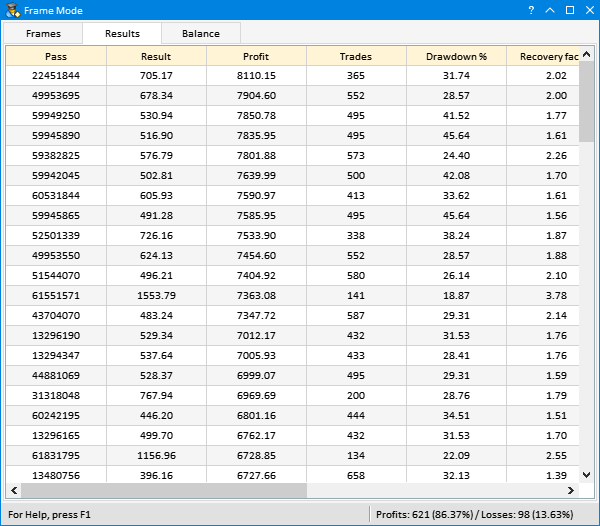Fig. 3. The table of optimization results in the graphical interface.

The user can view multi-symbol balance values of results from this table. If you select any table row, the custom event ON_CLICK_LIST_ITEM with the table identifier is generated. This allows determining the table, from which the message was received (provided there are several tables). The first column stores the pass number, so we can get the result data by passing this number to the CFrameGenerator::GetFrameData() method.

```//+------------------------------------------------------------------+
//| Event handler                                                    |
//+------------------------------------------------------------------+
void CProgram::OnEvent(const int id,const long &lparam,const double &dparam,const string &sparam)
{
//--- Event of clicking on table rows
if(id==CHARTEVENT_CUSTOM+ON_CLICK_LIST_ITEM)
{
if(lparam==m_table_param.Id())
{
//--- Get the pass number from the table
ulong pass=(ulong)m_table_param.GetValue(0,m_table_param.SelectedItem());
//--- Get data based on the pass number
m_frame_gen.GetFrameData(pass);
}
//---
return;
}
...
}
```

Each time the user selects a row in the table, the graph of multi-symbol balances is refreshed in the Balance tab: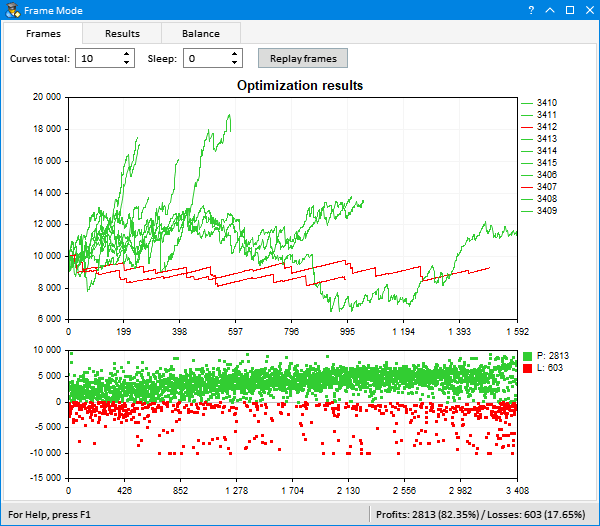Fig. 4. Demonstration of the obtained result.

We have got a useful tool enabling fast view of multi-symbol testing results.

### Conclusions

I have shown one more possible way of how you can work with optimization results. This topic is not yet completely studied and should be developed further. The GUI creation library allows creation of a wide variety of interesting and convenient solutions. You are welcome to suggest your ideas in comments to this article. Perhaps, one of the following articles will describe the optimization result processing tool you need.

Below, you can download the files for testing and detailed study of the code provided in the article.

File name Comment
MacdSampleMSFrames.mq5 Modified EA from the standard delivery - MACD Sample
Program.mqh File with the program class
CreateGUI.mqh File implementing methods from the program class in Program.mqh file
Strategy.mqh File with the modified MACD Sample strategy class (multi-symbol version)
FormatString.mqh File with auxiliary functions for strings formatting
FrameGenerator.mqh File with a class for working with optimization results.

Translated from Russian by MetaQuotes Software Corp.
Original article: https://www.mql5.com/ru/articles/4562

Attached files |
Experts.zip (23.7 KB)

#### Other articles by this author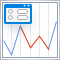Developing the oscillator-based ZigZag indicator. Example of executing a requirements specification
The article demonstrates the development of the ZigZag indicator in accordance with one of the sample specifications described in the article "How to prepare Requirements Specification when ordering an indicator". The indicator is built by extreme values defined using an oscillator. There is an ability to use one of five oscillators: WPR, CCI, Chaikin, RSI or Stochastic Oscillator.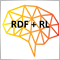Random Decision Forest in Reinforcement learning
Random Forest (RF) with the use of bagging is one of the most powerful machine learning methods, which is slightly inferior to gradient boosting. This article attempts to develop a self-learning trading system that makes decisions based on the experience gained from interaction with the market.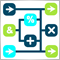Visual strategy builder. Creating trading robots without programming
This article presents a visual strategy builder. It is shown how any user can create trading robots and utilities without programming. Created Expert Advisors are fully functional and can be tested in the strategy tester, optimized in the cloud or executed live on real time charts.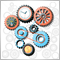Developing multi-module Expert Advisors
MQL programming language allows implementing the concept of modular development of trading strategies. The article shows an example of developing a multi-module Expert Advisor consisting of separately compiled file modules.